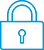cancel
Showing results for
Did you mean:It has been a while since anyone has replied. Simply ask a new question if you would like to start the discussion again.
New member
1 0 0 0
Message 1 of 3
888
Flag PostHP Recommended

Solved!

# Bug plotting cube root of x^2

Hp 50g
Other

Hi folks! Here is my first post here in Hp support forum.

I have a problem with my HP 50g graphic calculator.

When I try plotting a function like the cube root of x^2 i get only a graph for x>0 when there should be also a specular one for x<0.

When I try using the command SQ(x) instead of x^2 inside the cube root I get the right graph.

I think the command x^2 doesn't works so well inside cube roots. Is there a way to fix this?

Tiberio

Tags (2)
2 REPLIES 2
Level 9
970 953 119 185
Message 2 of 3
867
Flag PostHP Recommended

Solved!

## Bug plotting cube root of x^2

Hi,

Welcome to the forum.

The problem is that the 50g handles the equation slightly differently when using X^2 inside the cube root - i.e. it evaluates to X^(2/3).

When using the dedicated root and square functions, the 50g evaluates the square and root separately.

Try the following: plot (X^2)^(1/3) and you will see both the negative and positive side of the graph - because you are now forcing the 50g to again evaluate the ^2 and ^(1/3) parts separately.

The 50g is an immensely powerful machine, but it does have some quirks to get used to. However, as you learn to use it, these "quirks" actually can be useful and make it the powerhouse that it is.

Best regards.

-Bart
_________________________________________________________
calculator enthusiast
Tags (2)
Level 9
970 953 119 185
Message 3 of 3
784
Flag PostHP Recommended

Solved!

## Bug plotting cube root of x^2

Hi,

Some further thoughts on this.

I think the word "evaluate" was an unfortunate choice of words on my part - I should rather say "interpret".

I was not referring to the EVAL function which does some other manipulation too.

Enter XROOT(3,X^2) and EVAL will give you XROOT(3,X)^2

Interestingly if you enter XROOT(3,X)^2 as the graphing equation, it will show both positive and negative sides of the graph.

Also to note:
The XROOT function will find the real cube roots of negative numbers.

The power function will find only the complex cube roots of negative numbers (and hence not plot them).

So in the graphing equation:
-  XROOT(3,X^2) is interpreted as X^(2/3) and the power function will find only the complex cube roots of negative numbers (and hence not plot them).

-  XROOT(3,X)^2 probably uses the XROOT function and finds the real cube roots of negative numbers.

Further to this:
If you want the equation to be used in a specific order, you can write a program (yes, programs can be graphed 🙂 )
For example:
<< X ^ 2 1 3 / ^ >>
will ensure X is first to the power 2, then to the power 1/3
OR
<< X ^ 2 3 XROOT >>
will ensure X is first to the power 2, then cube root.

Let's say you store this program as AAA

Then in the graph equation let Y(1)=AAA

(NOTE: the program assumes the calculator is in RPN mode)

Regards,
Bart

-Bart
_________________________________________________________
calculator enthusiast
† The opinions expressed above are the personal opinions of the authors, not of HP. By using this site, you accept the Terms of Use and Rules of Participation